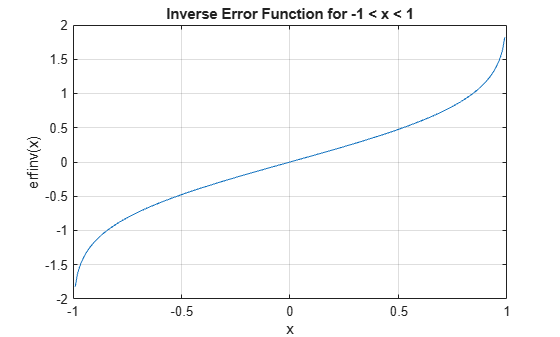# erfinv

Inverse error function

## Syntax

``erfinv(x)``

## Description

example

````erfinv(x)` returns the Inverse Error Function evaluated for each element of `x`. For inputs outside the interval `[-1 1]`, `erfinv` returns `NaN`. ```

## Examples

collapse all

`erfinv(0.25)`
```ans = 0.2253 ```

For inputs outside `[-1,1]`, `erfinv` returns `NaN`. For `-1` and `1`, `erfinv` returns `-Inf` and `Inf`, respectively.

`erfinv([-2 -1 1 2])`
```ans = 1×4 NaN -Inf Inf NaN ```

Find the inverse error function of the elements of a matrix.

```M = [0 -0.5; 0.9 -0.2]; erfinv(M)```
```ans = 2×2 0 -0.4769 1.1631 -0.1791 ```

Plot the inverse error function for -1 < x < 1.

```x = -1:0.01:1; y = erfinv(x); plot(x,y) grid on xlabel('x') ylabel('erfinv(x)') title('Inverse Error Function for -1 < x < 1')```Generate Gaussian distributed random numbers using uniformly distributed random numbers. To convert a uniformly distributed random number $x$ to a Gaussian distributed random number $y$, use the transform

`$y=\sqrt{2}er{f}^{-1}\left(x\right).$`

Note that because `x` has the form `-1 + 2*rand(1,10000)`, you can improve accuracy by using `erfcinv` instead of `erfinv`. For details, see Tips.

Generate 10,000 uniformly distributed random numbers on the interval `[-1,1]`. Transform them into Gaussian distributed random numbers. Show that the numbers follow the form of the Gaussian distribution using a histogram plot.

```rng('default') x = -1 + 2*rand(1,10000); y = sqrt(2)*erfinv(x); h = histogram(y);```

## Input Arguments

collapse all

Input, specified as a real number, or a vector, matrix, or multidimensional array of real numbers. `x` cannot be sparse.

Data Types: `single` | `double`

collapse all

### Inverse Error Function

The inverse error function erfinv is defined as the inverse of the error function, such that

`$\text{erfinv}\left(\text{erf}\left(x\right)\right)=x.$`

## Tips

• For expressions of the form `erfinv(1-x)`, use the complementary inverse error function `erfcinv` instead. This substitution maintains accuracy. When `x` is close to `1`, then `1 - x` is a small number and may be rounded down to `0`. Instead, replace `erfinv(1-x)` with `erfcinv(x)`.

## Version History

Introduced before R2006a